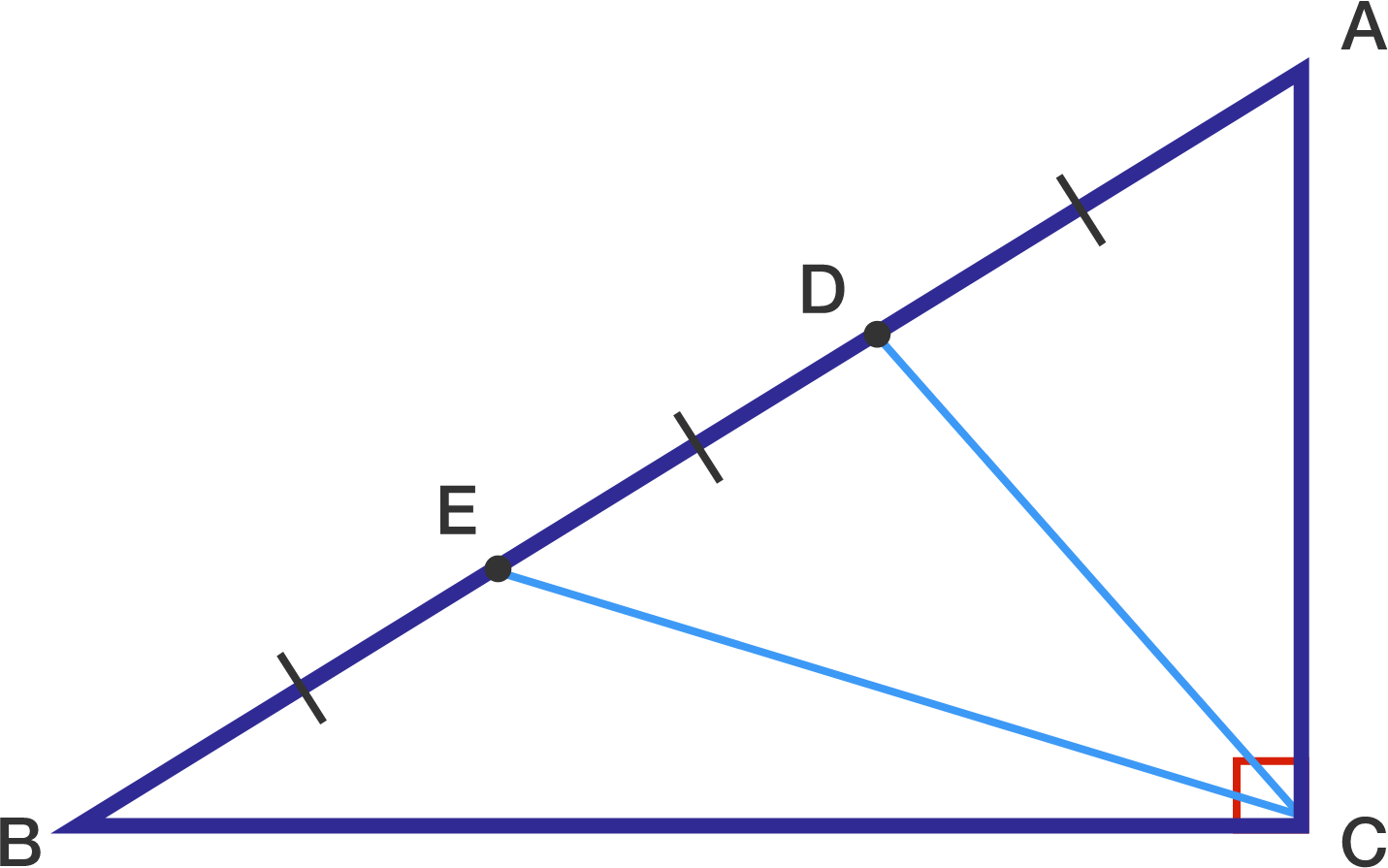# Trigonometric Lengths

Geometry Level 3

In triangle $ABC$, $\angle ACB = 90^\circ$. Points $A, D, E,$ and $B$ are consecutive points on side $AB$ such that $\overline{AD}=\overline{DE}=\overline{EB}$. If there exists $\theta$ such that $\overline{CD} = 5 \cos \theta$ and $\overline{CE} = 5 \sin \theta$, what is $\overline{AB}^2?$×